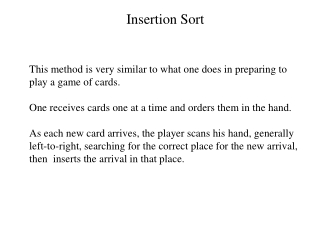DownloadDownload PresentationInsertion Sort

Insertion Sort

Télécharger la présentationInsertion Sort

- - - - - - - - - - - - - - - - - - - - - - - - - - - E N D - - - - - - - - - - - - - - - - - - - - - - - - - - -
Presentation Transcript

1. Insertion Sort This method is very similar to what one does in preparing to play a game of cards. One receives cards one at a time and orders them in the hand. As each new card arrives, the player scans his hand, generally left-to-right, searching for the correct place for the new arrival, then inserts the arrival in that place.

2. Insertion Sort • Efficient for small arrays • Given an array A with n elements, start with A as ”sorted” • Insert A into correct position with respect to A and A • Keep inserting until all n elements are sorted.

3. Insertion Sort 25 48 57 37 12 92 86 33 25 57 48 37 12 92 86 33 25 37 48 57 12 92 86 33 25 57 48 37 12 92 86 33 57 Inserted 48 Inserted 37 Inserted Initial Array

4. Insertion Sort • Assume an N-element array called A already exists, with K < N • elements in ascending order already in the first K locations.. • To placea new arrival: • Search each sequentially through the array until a key is found which • is greater than that of the new arrival. Call its location J. • Make space for the new arrival by moving the contents of A(J) • to A(J+1), A(J+1) to A(J+2), .......... • Insert the newly arrived element at A(J).

5. Insertion Sort void Insertion (int data[], int arraySize) { int i, j, tmp; for (i = 1; i < arraySize; i++) { tmp= data[i]; j = i; while (j > 0 && tmp < data[j -1] ) // move down 1position all // elements greater than tmp { data[j] = data[j -1]; j--; } data[j] = tmp; // insert tmp at proper position } }

6. O Notation If the initial file is sorted, only one comparison is made on each pass, so that the sort is O(n). If the file is initially sorted in the reverse order, the sort is O(n2), since the total number of comparisons is (n-1) + (n-2) + .................+3+2+1 = (n -1) * n/2 which is O(n2) However, the simple insertion sort is still better than bubble sort. The closer the file is to sorted order, the more efficient the simple insertion sort becomes. The average number of comparisons in thesimple insertion sort is also O(n2). The space requirements for the sort consist of only one temporary variable, temp.

7. Radix Sorting Radix sorting - also known as digit, pocket, and bucket orting. Radix sorts were used extensively in the punched card era to sort cards on electronic accounting machines (EAMs). This sort is based on the values of the actual digits in the positional representations of the numbers being sorted. Forexample, the number 345 in decimal notation iswritten with a 3 in the hundreds position, a 4 in the tens position, and a 5 in the units position.

8. Radix Sorting In a radixsorting, each pass through the list orders the data one digit at a time. The first pass orders the data on the units (least significant) digit. The second pass orders the data on the tens digit. The third pass orders the data on thehundreds digit, and so forth until the list is completely sorted by sorting thedata on the most significant digit.

9. 4132 2176 6456 2130 1466 3212 1455 2119 Radix Sort Unsorted xxx0 xxx2 xxx5 xxx6 xxx9 2130 4132 3212 1455 1st pass 2176 6456 1466 2119 xx1x xx3x xx5x xx6x xx7x 2130 4132 2176 2nd pass 3212 2119 1455 6456 1466 x1xx x2xx x4xx 1455 6456 1466 2119 2130 4132 2176 3212 3rd pass 3xxx 4xxx 6xxx 1xxx 2xxx 4th pass 1455 1466 2119 2130 2176 3212 4132 6456 1455 1466 2119 2130 2176 3212 4132 6456 Sorted

10. Radix Sorting If you analyze this sort, you will see that there are k sort passes, where k is the number of digits in the key. For each sort pass, we have n operations, where n is the number of elements to be sorted. This gives us the efficiency of kn, which in big-O notation is O(n). It is one of the best sorting techniques.